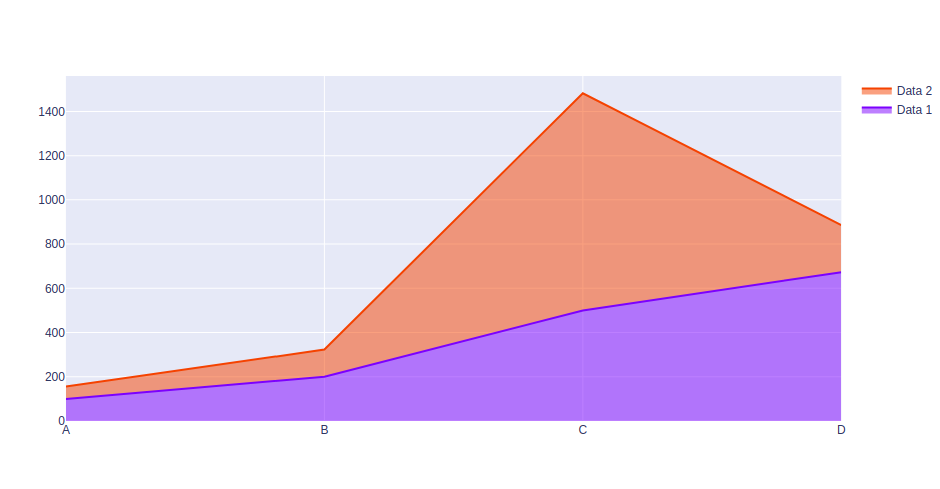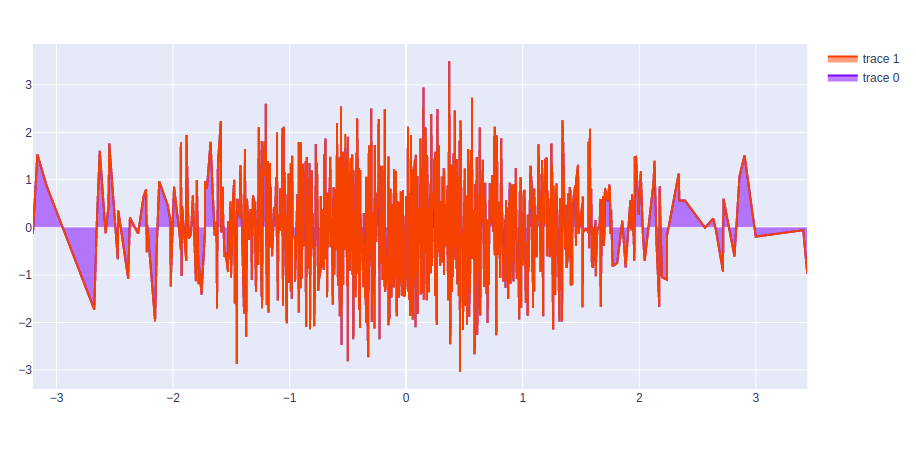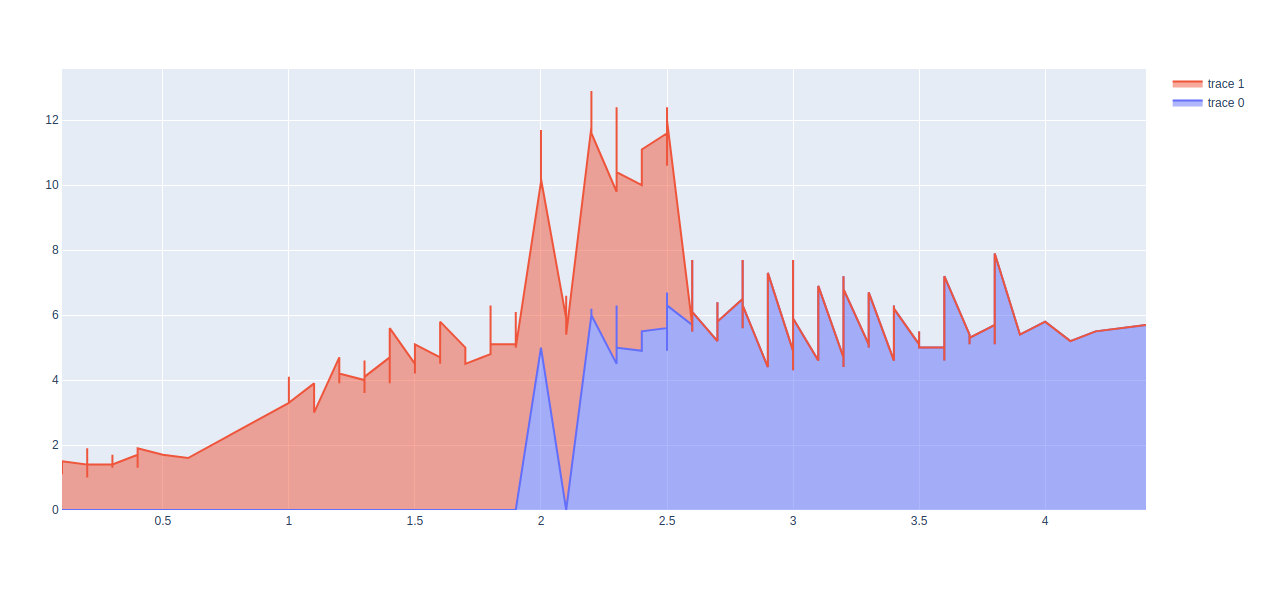Open in App
Not now

# How to Create Stacked area plot using Plotly in Python?

• Last Updated : 10 Jul, 2020

Plotly is a Python library that is used to design graphs, especially interactive graphs. It can plot various graphs and charts like histogram, barplot, boxplot, spreadplot, and many more. It is mainly used in data analysis as well as financial analysis. plotly is an interactive visualization library.

## Stacked Area plot

A stacked area chart is the amplification of a basic area chart to display the enlargement of the value of several groups on the same graphic. The values of each group are displayed on top of each other. This is the best chart to be used to show the distribution of categories as parts of a whole area where the cumulative total is unnecessary. It can be created by passing the stackgroup  parameter  in the scatter() method of graph_objects class

Example 1:

## Python3

 `import` `plotly.graph_objects as px``import` `numpy`` ` ` ` `# creating random data through randomint ``# function of numpy.random ``np.random.seed(``42``)`` ` `random_x``=` `np.random.randint(``1``,``101``,``100``) ``random_y``=` `np.random.randint(``1``,``101``,``100``)`` ` `x ``=` `[``'A'``, ``'B'``, ``'C'``, ``'D'``]`` ` `plot ``=` `px.Figure()`` ` `plot.add_trace(go.Scatter(``    ``name ``=` `'Data 1'``,``    ``x ``=` `x,``    ``y ``=` `[``100``, ``200``, ``500``, ``673``],``    ``stackgroup``=``'one'``   ``))`` ` `plot.add_trace(go.Scatter(``    ``name ``=` `'Data 2'``,``    ``x ``=` `x,``    ``y ``=` `[``56``, ``123``, ``982``, ``213``],``    ``stackgroup``=``'one'``   ``)``)`` ` `plot.show()`

Output:Example 2:

## Python3

 `import` `plotly.graph_objects as go``import` `numpy`` ` `  ` `plot ``=` `go.Figure(data``=``[go.Scatter(``    ``x ``=` `np.random.randn(``1000``),``    ``y ``=` `np.random.randn(``1000``),``    ``stackgroup``=``'one'``),``                       ``go.Scatter(``    ``x ``=` `np.random.randn(``10``),``    ``y ``=` `np.random.randn(``50``),``    ``stackgroup``=``'one'``)``])``                  ` `plot.show()`

Output:Example 3:

## Python3

 `import` `plotly.graph_objects as go``import` `plotly.express as px``import` `numpy`` ` ` ` `df ``=` `px.data.iris()`` ` `plot ``=` `go.Figure(data``=``[go.Scatter(``    ``x ``=` `df[``'sepal_width'``],``    ``y ``=` `df[``'sepal_length'``],``    ``stackgroup``=``'one'``),``                       ``go.Scatter(``    ``x ``=` `df[``'petal_width'``],``    ``y ``=` `df[``'petal_length'``],``    ``stackgroup``=``'one'``)``])``                  ` `plot.show()`

Output:My Personal Notes arrow_drop_up